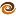# EmptyCrate

#### About Me | Contact Me | Training |ChaiScript.com | YouTube Channel

A comment was made on my YouTube channel that destructuring in C++17 would be much more useful if it worked with standard containers. At the moment I responsed that this would be someone impossible to get right without adding too much overhead.

After more thought, however, I started thinking maybe it would be.

What if we could do:

``````std::vector<int> vec{1,2,3};
auto [x,y,z] = vec;
``````

This could actually be handy. But convincing the language itself to do this would be hard, to say the least. How would it know what operations to perform on which containers? And is this something we really want built into the language?

An adapter of some sort could be employed to perform the transformation from a container into a list of elements that can be destructured by the language. So perhaps we end up with:

``````std::vector<int> v{1,2,3};
auto [x,y,z] = destructure<3>(v);
``````

We have to tell the compiler how many elements we want (otherwise, how would it know?). The rest can be automatically deduced. The type of container and the contained types.

Here is one option that works (note that this requires at least clang 4, which isn’t officially released at the time of this writing).

``````template<typename T, size_t ... S>
auto destructure_impl(T &t, std::index_sequence<S...>)
{
return std::forward_as_tuple(*std::next(std::begin(t), S)...);
}

template<size_t Count, typename T>
auto destructure(T &t)
{
return destructure_impl(t, std::make_index_sequence<Count>());
}
``````

We use `std::forward_as_tuple` which takes a set of parameters and generates a tuple of references to the values, so we end up with something like `std::tuple<int &, int &, int &>` being returned from our `destructure` function.

We can further extend it to do an offset and length:

``````template<size_t Start, typename T, size_t ... S>
auto destructure_impl(T &t, std::index_sequence<S...>)
{
return std::forward_as_tuple(*std::next(std::begin(t), Start+S)...);
}

template<size_t Start, size_t Count, typename T>
auto destructure(T &t)
{
return destructure_impl<Start>(t, std::make_index_sequence<Count>());
}
``````

So a complete example becomes:

``````#include <cstdint>
#include <array>
#include <vector>
#include <iterator>
#include <functional>

template<typename T, size_t ... S>
auto destructure_impl(T &t, std::index_sequence<S...>)
{
return std::forward_as_tuple(*std::next(std::begin(t), S)...);
}

template<size_t Count, typename T>
auto destructure(T &t)
{
return destructure_impl(t, std::make_index_sequence<Count>());
}

template<size_t Start, typename T, size_t ... S>
auto destructure_impl(T &t, std::index_sequence<S...>)
{
return std::forward_as_tuple(*std::next(std::begin(t), Start+S)...);
}

template<size_t Start, size_t Count, typename T>
auto destructure(T &t)
{
return destructure_impl<Start>(t, std::make_index_sequence<Count>());
}

int main()
{
std::vector<int> v{1,2,3};
auto [x,y] = destructure<1,2>(v);
x = 3;
y = 12;
return v;
}
``````

Which compiles down to

``````main:                                   # @main
pushq   %rax
movl    \$12, %edi
callq   _Znwm
movq    %rax, %rdi
callq   _ZdlPv
movl    \$3, %eax
popq    %rcx
retq
``````

This looks pretty good, but how does it compare to doing it by hand?

``````#include <cstdint>
#include <array>
#include <vector>
#include <iterator>

int main()
{
std::vector<int> v{1,2,3};
v = 3;
v = 12;
return v;
}
``````

Compiled:

``````main:                                   # @main
pushq   %rax
movl    \$12, %edi
callq   _Znwm
movq    %rax, %rdi
callq   _ZdlPv
movl    \$3, %eax
popq    %rcx
retq
``````

Which is… exactly the same. So we’ve accomplished some level of destructuring support for dynamic containers with 0 extra overhead. Is this something that we should propose for the C++20 standard library?

My Videos

Related